# Surface Area of a Cube

Companies package items in boxes use surface area to determine how much cardboard would be needed to make the box. This is important for determining the amount they need to make the boxes and in determining the cost.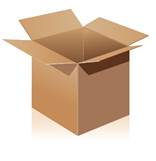In order to calculate the surface area, we need to include the area of every side of the box. Each side of a cube happens to be a square that is the same as each of the other squares used to make the cube.This makes calculating the surface area very simple. Determine the area of one of the squares using the formula A = s2. Then multiply by 6 because there are 6 congruent sides.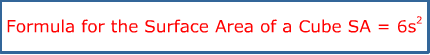Let's try it out!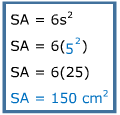Be sure to complete the exponent before multiplying by 6!

The area of the blue cube is 150 square centimeters.

Example:

Determine the surface area of the cube.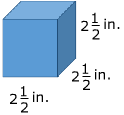Solution: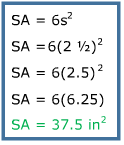If working with fraction is difficult for you, convert to a decimal!

The surface area of this cube is 37.5 square inches.

If you know the surface area you can also work backwards to determine the side lengths.

Example: The surface area of a cube is 86.64 square meters. Determine the side length of the cube.

Solution: Because we already know the surface area, we can work backwards through the steps to determine the length of the side.

The side length was squared and then multiplied by 6. So we will divide by 6 to undo the multiplying. Then we will take the square root to undo the squaring.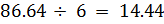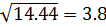Therefore, the sides of the cube are each 3.8 meters long.

Example: The surface area of a cube is 384 m2. Determine the volume of the cube. Solution: We need to know the side length in order to determine the volume. So the first step is to work backwards from the surface area of the side length.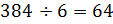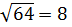The side length of the cube is 8 meters.

Now use this value to determine the volume using the formula V = s3.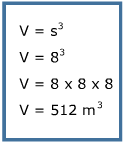The volume of the cube is 512 cubic meters.

Let's Review

To determine the surface area of a cube, calculate the area of one of the square sides, then multiply by 6 because there are 6 sides. This is the same as solving using the formula SA = 6s2. If you are given the surface area, you can determine the side length by working backwards. Take the surface area and work backwards by dividing by 6 and then take the square root.

 Related Links: Math Geometry Volume of a Cube Volume of Sphere

To link to this Surface Area of a Cube page, copy the following code to your site: